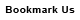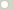# newton meter (Torque, Metric)

## 1 newton meter is equal to:Metric newton centimeter 100 dyne meter 100000 dyne centimeter 10000000 kilogram-force meter 0.10197162 kilogram-force centimeter 10.197162 gram-force meter 101.97162 gram-force centimeter 10197.162 British and U.S. long ton-force foot 0.00032926882 short ton-force foot 0.00036878107 pound-force foot 0.73756215 pound-force inch 8.8507458 ounce-force inch 141.61193ConversionsAreaCapacity and VolumeCircular measureComputer storageCurrencyDistance and LengthEnergy and WorkFuel ConsumptionMass and WeightPowerPressureSpeedTemperatureTimeTorqueSearchLink to us